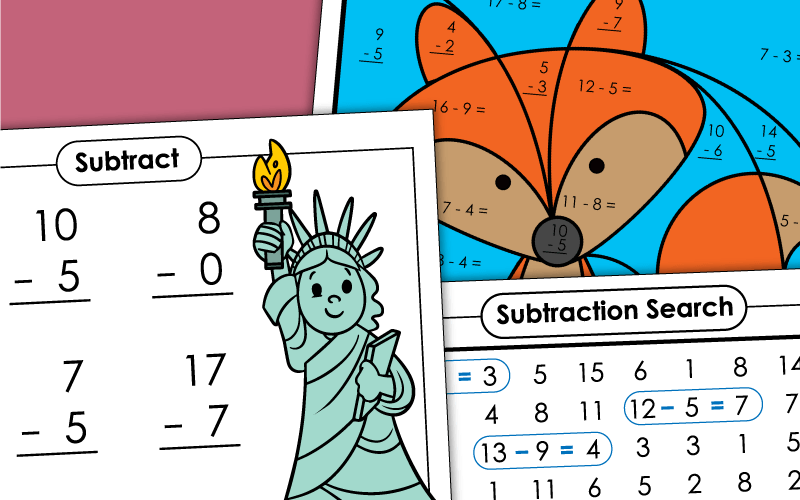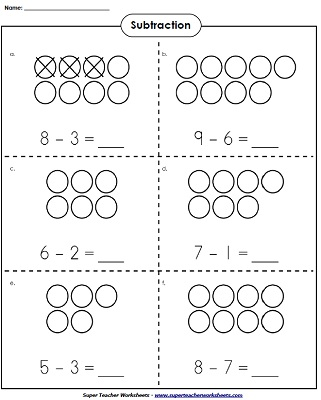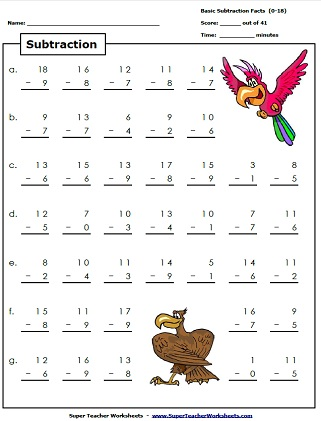# Basic Subtraction Worksheets## Games & Activities

Match each subtraction with with the answer in this fun card game.
Cut apart the stars along the dotted lines into 30 pieces. Spread out the pieces on a table and combine matching problems and numbers to make stars.
When students play this game, they will take turns rolling a die. The number they roll corresponds with a column on the game board. They must read and answer all subtraction facts in the column as quickly as they can.
Play Bingo and learn basic subtraction facts at the same time! This printable includes 42 calling cards with subtraction facts on them and a class set (30) of bingo boards.

## Concept Worksheets

Complete the picture models to help find the answer to the subtraction problems. Then write a subtraction fact for each.
Draw the counters in the space to provided to help form the number sentence for the subtraction problems.
Find and draw the missing counters and then complete the subtraction fact.
Have your students solve the subtraction word problem by drawing a picture to help find the answer.
This worksheet uses pictures of eggs to help students solve the subtraction problems and then write the number sentence to go with it.

## Cut-and-Glue Worksheets

Cut out ten number tiles at the bottom of the page. Glue each number next to the correct subtraction fact.
Print out these subtraction puzzle pieces for a fun way to practice basic subtraction facts.

## Number LineSubtraction

Determine which subtraction problems are being shown by each of the number lines.
Use the number line to determine the missing number in each subtraction fact.
Use the number lines to determine the missing quantity in each subtraction fact.  Use the "count back" technique.
Students use the number line to help them subtract.

## Subtraction Fact Practice

Write the answers to the subtraction facts on the caterpillar. All facts have minuends of 20 or less. (example: 15-5)
Some of these subtraction facts have correct answers and some are incorrect. Circle the correct answers and cross out the incorrect ones.
Students can hunt for basic subtraction facts with this printable number puzzle. Find and circle all 20 subtraction facts.
Help the rocket find the star. Along the way, answer the subtraction facts with minuends to 20 and answers to 10.

## Mystery Pictures

Solve the basic subtraction problems (all minuends are 18 or less; all subtrahends are exactly 9). Then color the mystery scene according to the key. Scene will show a beach ball and sand pail.
Solve the subtraction problems and color-by-number to reveal a picture of a colorful parrot.
Solve the subtraction facts and color according to the key to reveal a fox!
Color the robot picture by answering the subtraction facts and using the key.
Subtraction fact blast off!  Solve the facts and color the rocket.
Solve the basic subtraction problems and color-by-number to reveal a picture of a rooster.
Find the differences and color the dog picture according to the color code.
Write the answers to the subtraction facts and color the royal castle.
Write the answers to the subtraction problems, then color the mystery picture of a clownfish.
A colorful hot air balloon will be revealed when you answer the math facts and color according to the key.
Find the differences for each fact shown. The color the spaces to reveal a picture of a ladybug landing on a purple flower.
When they solve and color the picture, students will see a colorful sailboat.
Find the difference and write them in the correct sections of the picture. Then color according to the key to reveal a beautiful butterfly.

## Basic SubtractionWorksheet Generator

Basic Subtraction Worksheet Generator

Create your own custom subtraction worksheets with basic facts. You choose the range for the subtrahends and the differences. This generator tool lets you to create worksheets with 25 or 50 problems.

## Subtraction Fact Practice

Print out this worksheet to practice basic subtraction facts with minuends up to 20.
Download this basic subtraction activity and practice subtraction facts with minuends up to 20.
Use this printable math activity to help children practice basic subtraction facts with minuends up to 20.
This worksheet has 20 subtraction facts to solve with minuends up to 20.
Have your students solve these basic subtraction facts on this printable patriotic-themed worksheet.
This printable subtraction worksheet has twenty horizontal subtraction facts to be solved with minuends up to 20.

## Subtraction Speed Drills

This worksheet has 43 basic subtraction facts. How quickly can you students solve them? All numbers are 18 or less. Differences are all equal to or less than nine.
Solve 43 basic subtraction problems as quickly as possible. All numbers are 20 or less. Differences are ten or less.
Solve all of the mixed addition and subtraction facts as quickly as possible. Includes sums up to and including 20 and differences up to and including 10.

## Flashcards

Download and print flash cards for all basic subtraction facts with minuends to 20 and answers to 10. There are 9 cards per page.
A complete set of basic subtraction flashcards up to 18 - 9. This file has 4 large cards per page.

## Related Pages on Super Teacher Worksheets

Worksheets for two, three, and four-digit subtraction with and without regrouping

This page has a mixture of basic subtraction and basic addition facts. Includes practice worksheets, number lines, and flashcards.

## Pictures of OurSubtraction WorksheetsMy Account
Site Information